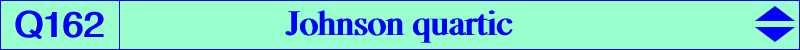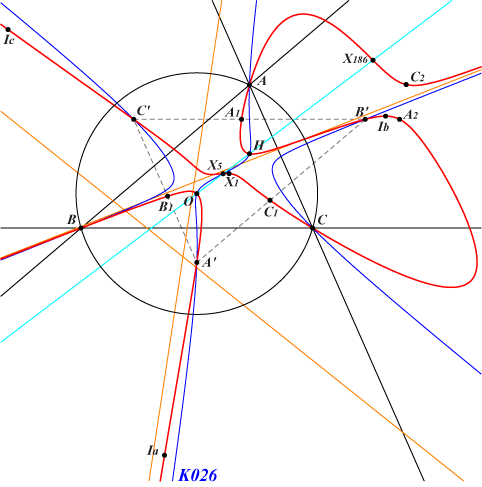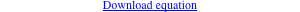too complicated to be written here. Click on the link to download a text file.X(1), X(3), X(4), X(5), X(186), X(523), X(847) excenters infinite points of K003 A', B', C' : vertices of the Johnson triangle, the reflections of A, B, C in X(5) foci of the MacBeath inconic (O, H and two imaginary points on the line X5-X523) A1, B1, C1 and A2, B2, C2 on the sidelines of the Johnson triangle and on the bisectors of ABCLet P be a point with circumcevian triangle PaPbPc. Denote by Na, Nb, Nc the nine points centers of triangles PaBC, PbCA, PcAB respectively. PaPbPc and NaNbNc are perspective if and only if P lies on the quartic Q162 (together with the circumcircle). The locus of the perspector is a very complicated curve of the 10th degree. Q162 shares 12 identified points with some known circum-cubics, namely : • K003 : A, B, C, 4 in/excenters, 3 points at infinity, X(3), X(4). • K005 : A, B, C, 4 in/excenters, X(3), X(4), X(5), 2 imaginary foci of the MacBeath inconic. • K026 : A, B, C, 3 points at infinity, X(3), X(4), X(5), vertices of the Johnson triangle. • K028 : A, B, C, 3 points at infinity, X(3), X(4) counted twice, X(5), 2 imaginary foci of the MacBeath inconic. • K049 : A, B, C (all three counted twice), 3 points at infinity, X(4), X(5), X(847). Indeed, the tangents at A, B, C to both curves concur at X(5). • K001 : A, B, C, 4 in/excenters, X(3), X(4) and three collinear points on the perpendicular at X(11563) to the Euler line. This latter example remains actually true for every cubic (K) of the Euler pencil with another perpendicular. More precisely, if P is the pivot defined by OP = S OH (vectors), then the perpendicular (L) intersects the Euler line at Q, the image of X(11563) under the homothety with center X(5), ratio 1 - 1/(2S). With K001, K003, K005 as above, we have S = ∞, 0, 1/2 hence Q = X(11563), X(30), X(5) respectively. This generates Q162 as the locus of the commun points of (K) and (L). See the analogous Q163, Q164 and Q165 where nine points centers X(5) are replaced by X(30), X(381), X(25) respectively. See Q023 for a generalization.